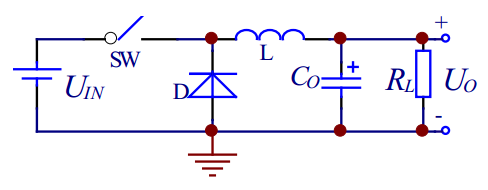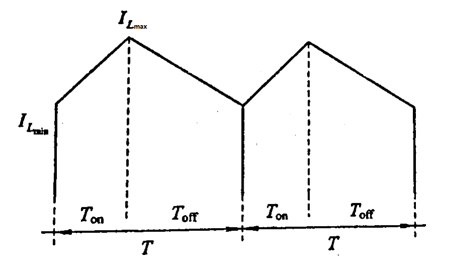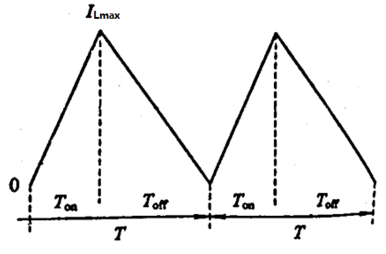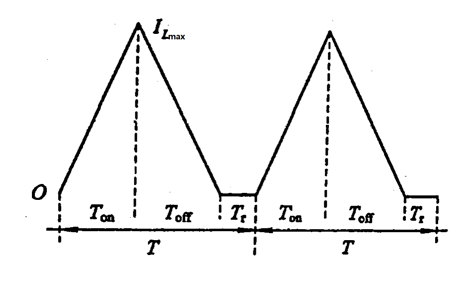# 开关电源学习笔记1 --- Buck变换器的基本原理

DC-DC变换器最基础的主要有三种：Buck变换器，Boost变换器和Buck-Boost变换器

Buck变换器：即降压变换器，其简单电路组成如下• 其中的开关SW一般使用功率三极管或功率MOS管，由PWM信号来控制SW高速进行开关
• 电感是降压的关键储能器件
• V D V_{D} 为续流二极管
• C O C_{O} 为输出滤波电容
• R L R_{L} 为负载电阻

（实际上就是L的充能过程,通过电感的电流会不断增加）
U = L d i d t U = L\frac{di}{dt} 得，电感两端的电流变化量为 U o n L t \frac{U_{on}}{L}t

Δ I \Delta I = U o n L T o n \frac{U_{on}}{L}T_{on} T o n T_{on} 为一个周期内开关的接通时间）

（实际上就是L的放能过程,通过电感的电流会不断减小）
U = L d i d t U = L\frac{di}{dt} 得，电感两端的电流变化量为 U o f f L t \frac{U_{off}}{L}t

Δ I \Delta I = U o f f L T o f f \frac{U_{off}}{L}T_{off} T o f f T_{off} 为一个周期内开关的断开时间）

”伏秒积“平衡

U o n L T o n \frac{U_{on}}{L}T_{on} = U o f f L T f f \frac{U_{off}}{L}T_{ff}

• 第一种是：开关断开后，电感电流尚未下降到零的时候，开关又被接通
这种模式称为CCM模式• 第二种是：开关断开后，电感电流刚好下降到零的时候，开关又被接通
这种模式称为BCM模式• 第三种是：开关断开后，电感电流下降到零之后，过了一段时间，开关才被接通
这种模式称为DCM模式1. 在CCM和BCM模式下
D = T o n T = T o n T o n + T o f f D = \frac{T_{on}}{T} = \frac{T_{on}}{T_{on} +T_{off}}
带入伏秒积： U o n T o n U_{on}T_{on} = U o f f T o f f U_{off}T_{off} ，得 D = U o f f U o n + U o f f = U o + U D U i n − U s w + U D = U o U i n D = \frac{U_{off}}{U_{on} +U_{off}} = \frac{U_{o} + U_{D}}{U_{in} - U_{sw} +U_{D}} = \frac{U_{o}}{U_{in}} （忽略二极管和开关管压降）
U o = D U i n U_{o} = DU_{in}
由于占空比D <= 1， U o < = U i n U_{o} <= U_{in} 这就完成了降压的功能
2. 同理，在DCM模式下，得出的结果依然是 U o < = U i n U_{o} <= U_{in}04-031万+
03-291万+
01-092008
05-25
08-03224
07-21211
02-28
02-2666
11-26121
05-181万+
01-133万+
05-10
03-21
01-081263
11-133721
03-144548